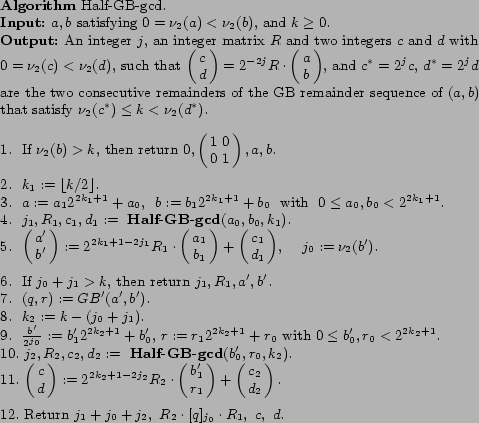# A Recursive Binary Gcd Algorithm

### Damien Stehlé and Paul Zimmermann

Abstract: The binary algorithm is a variant of the Euclidean algorithm that performs well in practice. We present a quasi-linear time recursive algorithm that computes the greatest common divisor of two integers by simulating a slightly modified version of the binary algorithm. The structure of our algorithm is very close to the one of the well-known Knuth-Schönhage fast gcd algorithm; although it does not improve on its $O(M(n) \log n)$ complexity, the description and the proof of correctness are significantly simpler in our case. This leads to a simplification of the implementation and to better running times.

Slides in French: ps.gz, pdf.

### Errata

We thank Dan Bernstein and Bo-Yin Yang for pointing out that Gn = 0,1,-1,5,-9,29,-65,181,-441,1165,..., which is the worst-case for Theorem 2, is not necessarily the worst-case for the algorithm (as claimed in Section 6.2). More details here. Also, in Theorem 3, the inputs that are claimed to reach the upper bound do not achieve that property as they are not even integers.

We thank Niels Möller for having pointed out to us a mistake in Figure 6 of the article. It should be:Each time algorithm Half-GB-gcd is called by algorithm Fast-GB-gcd, the parameter k is chosen as.

### Some Statistical Tests Related to the GB Division

Distribution of the quotient of GB(a,b) when a and b are chosen uniformly in [|1,2^10-1|] with nu_2(b) > nu_2(a). 10^6 samples. In percentages. Notice that quotients with the same bit length appear with equal frequencies.

 |q| 1 3 5 7 9 11 13 15 17 19 21 23 25 27 29 31 >32, <64 >64 mean 66.68 16.74 4.17 4.13 1.02 1.04 1.05 1.03 0.26 0.25 0.26 0.26 0.27 0.25 0.26 0.26 1.04 1.04

Average number of divisions in the GB Euclidean execution starting from a,b when a and b are chosen uniformly in [|1,2^n-1|] with nu_2(b) > nu_2(a). 10^3 samples if n<=100, 10 samples if n>=200.

 n 10 20 30 40 50 60 70 80 90 100 200 300 400 500 600 700 800 900 1000 mean 4.9 10 15.1 20.3 25.6 30.6 35.6 40.8 45.8 51 104 155 207 253 308 357 406 469 518 worst 10 18 26 31 35 44 50 60 65 67 117 162 226 271 324 385 445 480 546

Distribution of the quotients appearing in the GB Euclidean execution starting from a,b when a and b are chosen uniformly in [|1,2^n-1|] with nu_2(b) > nu_2(a). 1 sample. In percentages.

 n nbr of quotients |q|=1 3 5 7 9 11 13 15 >16 1000 491 64.6 18.9 3.9 4.1 1 0.6 1.2 0.4 <=5.0 2000 1029 67.5 15.9 3.3 3.7 1.3 1.6 0.8 1.7 <=4.2 3000 1588 66.8 17.3 4 3.7 1.6 1.4 0.8 0.8 <=3.6 4000 2085 68.1 16.1 4.3 3.9 1 0.9 1.1 0.9 <=3.7 5000 2593 66.6 17.1 4 4 1.1 1 1.1 1.1 <=4.0 6000 3058 67.0 15.7 4.3 4.4 1.4 0.8 1.1 0.9 <=4.4 7000 3613 65.8 17.5 4.4 4.6 1.2 0.6 0.9 1.1 <=3.9

These experimental results have been explained precisely by the average-case analysis of the GB Euclidean algorithm that has been undertaken by Daireaux, Maume-Deschamp and Vallée, in their article "The Lyapunov Tortoise and the Dyadic Hare".